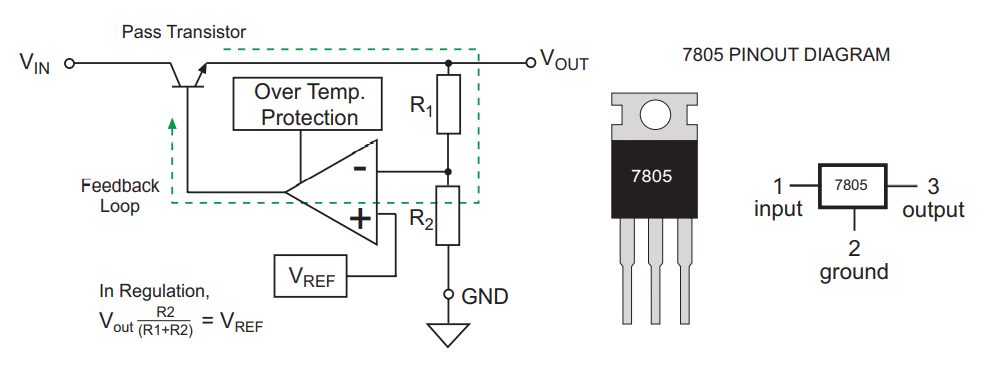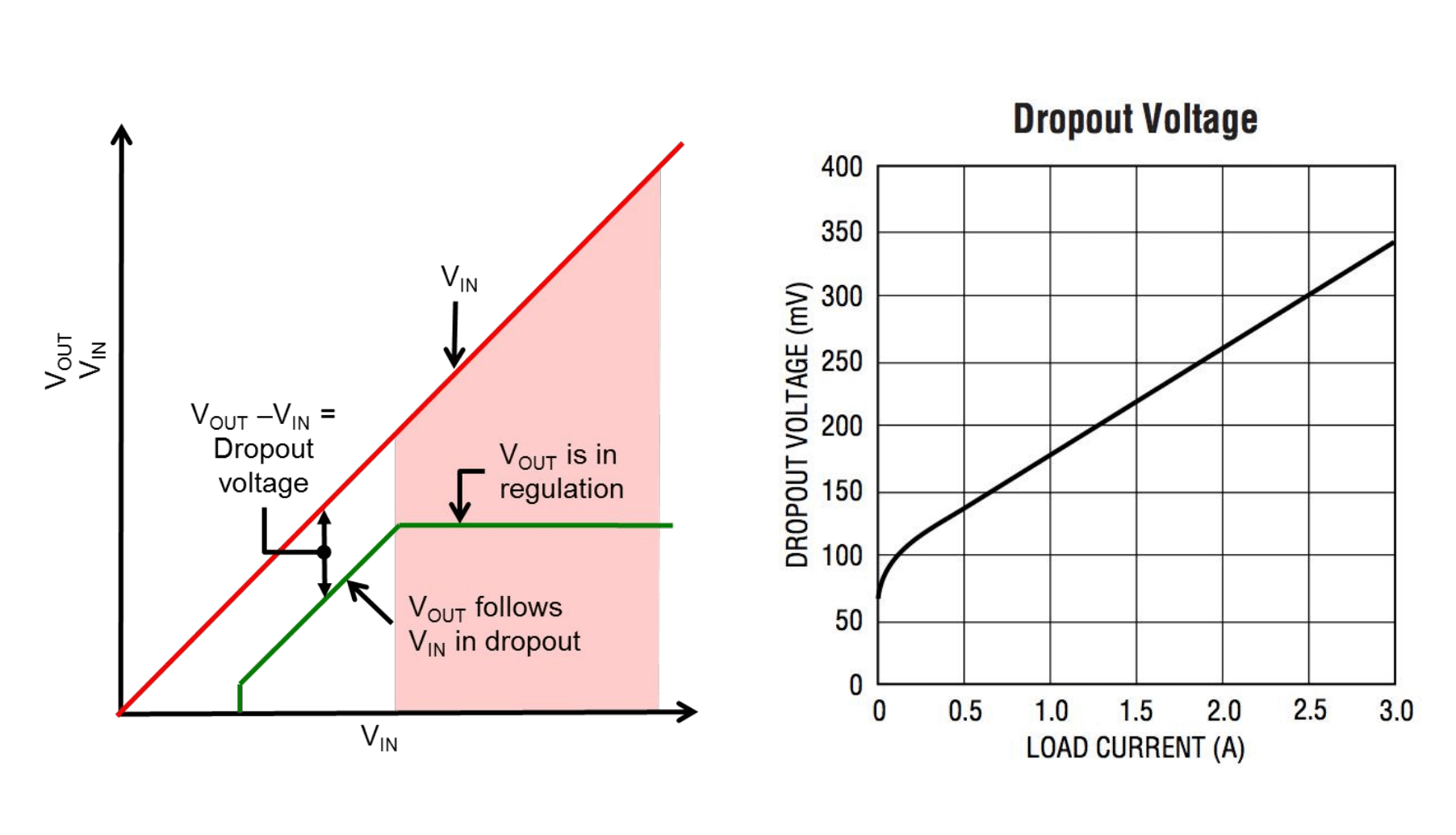# 电源拓扑 - 线性稳压## 线性稳压器的原理## 线性稳压器的效率

$η = \frac{P_{out}}{P_{in}}$

$P_{out}=V_{out}*I_{out},$
$P_{in}=V_{in}*I_{in},$
$I_{in}=I_{out}+I_{q}$

$$I_{q}$$ 是无负载下的静态电流。

## 优缺点及应用

• 电路简单、便宜
• 输出端噪声低
• 对噪声的隔离度高
• 快速的瞬态响应

### 缺点

• 需要一定的压差才能起稳压作用，因此只能做降压用。
• 在压差比较大的情况下，转换效率比较低，损耗都以发热的方式消耗掉，并影响板卡的稳定性、可靠性。
• 功率至上，即便负载电路并不需要很大电流。这样做的后果就是所有元件即便非必要的时候也工作在满负荷下，结果产生高很多的热量。
• 在压差不够的情况下，会出现比较大的纹波。

### 应用

• 对供电电源的噪声要求严格的模拟电路、时钟产生电路等。
• 小电流、电源转换效率影响不大的数字电路供电场景。